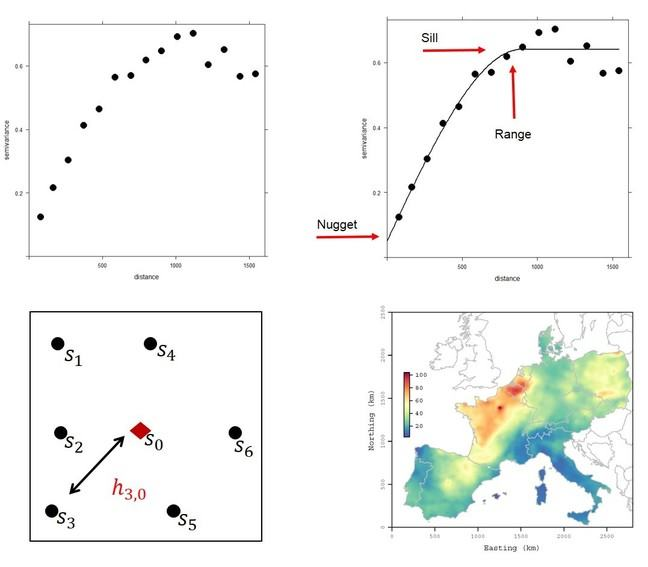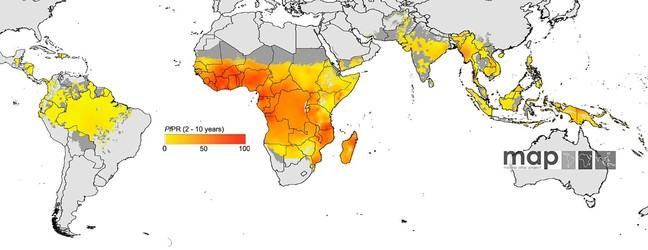## Want to keep learning?

This content is taken from the University of Twente's online course, Geohealth: Improving Public Health through Geographic Information. Join the course to learn more.
3.16

# The variogram

You were introduced to the concept of spatial dependence. In this step you will learn about the variogram (also referred to as the semi-variogram). A variogram is used to describe and model spatial dependence. The variogram is used widely in geostatistics. The equation for the variogram is:

$\hat \gamma(h) =\frac{1}{2n(h)} \sum_{i=1}^{n(h)}(y(s)-y(s+h))^2$

Let’s look at what this equation means. $$y$$ is the response variable (e.g., reflectance in an image, air pollution concentration, malaria parasite rate) which is taken at a specific location $$s$$. We calculate the difference in the attribute value between two observations measured at locations separated by a lag distance $$h$$ (hence ($$s$$+$$h$$)). Lag distance refers to the geographic separation between two observations. There are $$n(h)$$ pairs of points separated by $$h$$. We take the average of these squared differences for the $$n(h)$$ pairs. Note the 1/2 on the right-hand side of the equation. We multiply the average squared difference by 1/2 . Hence $$γ ̂(h)$$ is actually the semi-variance for pairs of points separated by $$h$$. For short lags we expect the average squared difference to be small (because measurements separated by short lags are expected to be similar). For large lags we expect the average squared difference to be larger. We can plot the semi-variances for different $$h$$’s against lag. This plot is called the sample semi-variogram or sample variogram (also called the experimental or empirical variogram).*Figure 1 (top left) An example sample variogram, (top right) Sample variogram with fitted model (curved line). The sill, nugget and range are indicated. (bottom left) Schematic diagram showing a location with no measurement (red diamond) and locations where measurements are taken (black dots). The arrow indicates the distance between two locations. (bottom right) Map of PM10 air pollution concentration across Europe, based on the data in Figure 1 (top right) of Step 3.15.

An example sample variogram is given in Figure 1 (top left). Note that the value of semi-variance increases with increasing lag distance. For short lag distances the attribute values are similar and the semi-variance is small. At large lag distances the attribute values are dissimilar and the semi-variance is large.

We can already gain useful information from the sample semi-variogram. The lag distance where the sample variogram flattens is called the range. This is the maximum spatial separation where we expect two points to be correlated. The range is associated with the variogram sill, which is the maximum variability in the data. Finally, the point where the variogram approaches the y-axis is the nugget. The nugget is the non-spatial variability. These three parameters (sill, nugget, range) can be identified if we fit a model to the sample variogram. The model is a curved line, as illustrated in Figure 1 (top right).

In Figure 1 (top right) the range occurs at approximately 900 m. Two observations separated by less than 900 m would be expected to be correlated whereas two observations separated by more than 900 m would be expected to be uncorrelated. Two observations separated by 300 m are expected to be more correlated than two observations separated by 500 m. The variogram model tells exactly how correlated are two observations that are separated by a given distance.

The sample variogram and variogram model are useful for exploring the spatial dependence in a dataset. They can also be used for mapping. Consider Figure 1 (bottom left). The red diamond indicates a location where we do not have a measurement whereas we do have measurements at the black dots. We know how far the red diamond is from each black dot. Using the variogram we can then say how correlated it is expected to be with that black dot. We can then predict the attribute at the red diamond as a weighted average of the attributes at the black dots – where the weights are based on the correlations. This prediction is also called interpolation. The geostatistical approach to prediction is often called kriging, named after Danie Krige, an early researcher and practitioner in geostatistics.

Figure 1 (bottom left) illustrates prediction at a single location. If we predict at multiple locations on a grid we can create a map. Examples are shown in Figure 1 (bottom right) and Figure 2, which are based on data presented in the step on Spatial Dependence.Figure 2 Map of malaria parasite rate, based on the data shown in Figure 3 of the Article on Spatial Dependence. See also Hay et al. 2009.

The maps shown in Figure 1 (bottom right) and Figure 2 are the concluding examples in the module on spatial statistics in this online course. These are important examples in the context of geohealth. Consider the air pollution example. We can use such maps to estimate individual exposure as part of an environmental epidemiological study into the health effects of air pollution. The malaria example is different because the disease itself is mapped rather than the possible cause of a disease. Such maps can be used to target interventions aimed at eliminating a disease (e.g., drug treatments, bed nets) and for evaluating the success of interventions.

References

Oliver, M.A., Webster, R. (2014) A tutorial guide to geostatistics: Computing and modelling variograms and kriging. CATENA 113, 56-69.

Hay, S. I., C. A. Guerra, P. W. Gething, A. P. Patil, A. J. Tatem, A. M. Noor, C. W. Kabaria, B. H. Manh, I. R. Elyazar, S. Brooker, D. L. Smith, R. A. Moyeed and R. W. Snow (2009). A world malaria map: Plasmodium falciparum endemicity in 2007. PLoS Medicine 6(3): e1000048. DOI: 10.1371/journal.pmed.1000048.